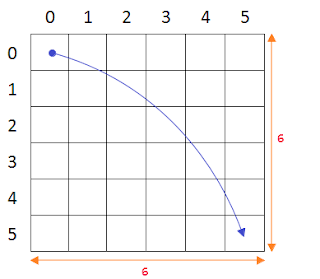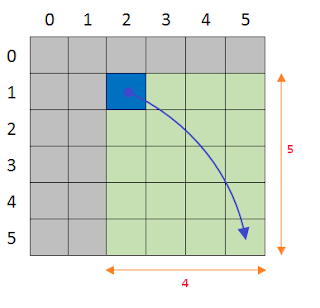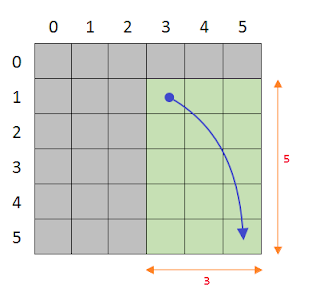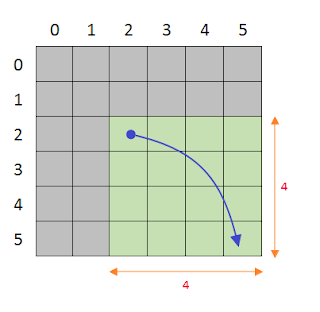# GATE2007-84

4.7k views

Suppose that a robot is placed on the Cartesian plane. At each step it is allowed to move either one unit up or one unit right, i.e., if it is at $(i,j)$ then it can move to either $(i + 1, j)$ or $(i,j + 1)$.

How many distinct paths are there for the robot to reach the point $(10,10)$ starting from the initial position $(0,0)$?

1. $^{20}\mathrm{C}_{10}$
2. $2^{20}$
3. $2^{10}$
4. None of the above

edited
0
I am not able to solve question 85 even by referring the solution many times. Kindly, explain in detail. U are asking to subtract some paths and u are multiplying. Plz help me.
15

Very nice explanation

0
It's the same thing that I did missed it by last step :( thanks though
5

from 9:20

In ques given, $r = \text{Move Right}$ and $u = \text{Move Up}$
so using ${10}$ combination of $r$ and ${10}$ combinations of $u$ moves we get a solution.

Convert the graphical moves to text and one such solution we get,

$= \{u, u, u, u, u, u, u, u, u, u, r, r, r, r, r, r, r, r, r, r\}$

now all possible arrangements of them is given by $= \frac{20!}{10! \times 10!} = {20 \choose 10}.$

Hence, option A is true.

edited
1

0

how are you getting option C

0
I'm not saying Option C. What I'm saying is, how we are exactly reducing no of choices, by breaking one of up-right link ! I think it might be because of there are lot of choices even after that link.. That may be it, Just was confused little bit !
0
Suppose if we are allowed to take 2 steps up and 2 steps right. Then we need 5 steps of U and 5 steps of R. Then the answer should be $\frac{20!}{5! * 5!}$.

Please correct me if I am wrong.
0
"Suppose if we are allowed to take 2 steps up and 2 steps right. Then we need 5 steps of U and 5 steps of R. Then the answer should be 20!/(5! * 5!)"

Is this approach given by @rohan mishra correct???
0
good explanation
1
It will be 10!/5!*5!
0

@amarVashishth sir nice explanation.

I approached this in similar manner .

As to reach diagonal elements we need both up moves and right moves as well ( and drawing for smaller example gives us clearer picture like from (0,0) to (1,1) or or till (2,2) )

Now from small example it is clear that we need equal amount of up and right moves and then we can finally apply the formula of possible arrangements (with repitition) and get the answer.

P.S.-> I know Guess work is a bad thing ,but after seeing option my mind was convinced that option (b) and (c) are not possible because of their large magnitude compared to first option.

This answer is not directly related to the QS But this may help to view this problem in a recursive way.How many ways we can traverse this grid from $(0,0)$ to $(5,5)$.

Allowed moves from a cell are:

1. Left to right by $1$ unit.
2. Top to Bottom by $1$ unit.

This problem has $O(1)$ solution.

But we will see how we can use top-down recursion and memoization to calculate that value.

Currently, we have a problem size of $(6,6)$ i.e we have still to cover a $6^*6$ grid and we are at cell $(0,0)$.Let's say we are at cell $(1,2)$ somehow.Now, this situation we have a $4^*5$ grid to traverse. What are the steps we can take in this cell or in this state ?

Well two things can be done.

1. Go right
2. Go down.If we move right, then we will have to traverse a smaller grid of size $3^*5$And if we move down, we will have to traverse a different smaller grid of size $4^*4$.So, what we have seen is that from a bigger size problem of $4^*5$ grid we can arrive at smaller grid size problem by applying two different steps.

And if we sum up the two smaller subproblem solution we can get the result of bigger problem solution. (which was of size $4^*5$ ).

Therefore  $\text{solution}(m,n) = \text{solution}(m-1,n) + \text{solution}(m,n-1)$, this is the recursion and the Top Down approach will seek for solution of $(m,n)$ without knowing the solution of $(m-1,n)$ and $(m.n-1)$. Using recursion we will recurse towards smaller and smaller sub problem untill we hit in some base case.

And the base here is:When we arrive at cell $(5,5)$. In this state, we have arrived at the destination and there nothing to do now, and how many ways to go from $(5,5)$ to $(5,5)$. It is equal to $1$ ( means don't move in this particular case).

Therefore  $\text{solution}(1,1)$ $= 1$.

To speed up the solution and not repeating same sub problem, again and again, we will use memoization table.

Here is the code:

#define MAX_X 6
#define MAX_Y 8
int dp[MAX_X+1][MAX_Y+1];
int solve(int m,int n) {
if(dp[m][n] != -1) return dp[m][n];
int res = 0;
if(m > 1) res += solve(m-1,n);
if(n > 1) res += solve(m,n-1);
return dp[m][n] = res;
}
int main() {
for(int i=0;i<(MAX_X+1);i++)
for(int j=0;j<(MAX_Y+1);j++)
dp[i][j] = -1;
dp = 1;
int m = MAX_X,n = MAX_Y;
printf("%d\n",solve(m,n));
return 0;
}
0

Superb. thanks :-)

0
ur explanations r damn too gd ....

84 At each move, robot can move either 1 unit right, or 1 unit up, and there will be 20 such moves required to reach (10,10) from (0,0). So we have to divide these 20 moves, numbered from 1 to 20, into 2 groups : right group and up group. Right group contains those moves in which we move right, and up group contains those moves in which we move up. Each group contains 10 elements each. So basically, we have to divide 20 things into 2 groups of 10 10 things each, which can be done in 20!/(10! ☓10!)=20C10 ways. So option (A) is correct.

edited by
0

This is unordered partition hence ,should not it be 20!/((10!*10!)*2!) ?

0
No, it is not because the order of steps in each group have to be specific. For e.g. an up move from y=0 to y=1 has to appear before the up move from y=1 to y=2 which in turn has to come before further up moves. Similarly it can be inferred for x moves.

Secondly, logic here is to place moves from each group in their specific order into the available 20 choices. Ordering of the groups themselves need not be considered.
The answer for 84 is A. It is same as selecting 10 out of 20 elements.
0

20C10 or 210?

0
It is 20 C 10.

@amarvashishth has given nice explanation, but if you want to visualize how it does work then draw 2x2 grid and try to draw all path from (0,0) to (2,2) you can easily see how it does work and you also can verify answer for 10X10here it give 4C2  =6  // { R,R,U,U} any path you follow you will encounter only two up and two right which is 4! /(2!*2!)

edited
In order to move from (0,0) to (10,10)there will be total 20 movement out of which there will be 10 right,10 upward movement combination

so 20!/10!.10!= c(20,10)

## Related questions

1
3k views
Suppose that a robot is placed on the Cartesian plane. At each step it is allowed to move either one unit up or one unit right, i.e., if it is at $(i,j)$ then it can move to either $(i + 1, j)$ or $(i,j + 1)$ ... $^{20}\mathrm{C}_{10} - ^{8}\mathrm{C}_{4}\times ^{11}\mathrm{C}_{5}$
What is the maximum number of different Boolean functions involving $n$ Boolean variables? $n^2$ $2^n$ $2^{2^n}$ $2^{n^2}$
Consider the following segment of C-code: int j, n; j = 1; while (j <= n) j = j * 2; The number of comparisons made in the execution of the loop for any $n > 0$ is: $\lceil \log_2n \rceil +1$ $n$ $\lceil \log_2n \rceil$ $\lfloor \log_2n \rfloor +1$
Consider the following program segment. Here $\text{R1, R2}$ and $\text{R3}$ ... interrupt occurs during the execution of the instruction INC R3 , what return address will be pushed on to the stack? $1005$ $1020$ $1024$ $1040$